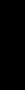FINANCIAL MANAGEMENT - MARKUP

Don’t confuse markup with profit. If you want a certain percentage of profit and you estimate your costs and add the desired profit percentage as markup, you’re making an error. Your profit should be a percentage of the selling price, not a markup on cost.

To determine markup (and profit):

• Deduct the desired profit percentage from 100 percent.
• Divide the desired profit percentage by this figure.
• Multiply your cost by the result. This will be your profit.

Example: Cost of job is \$100,000. Desired profit percentage is 15%. Subtract 15% from 100%. Balance is 85%. Divide 15% by 85%. Quotient is 17.65%. Multiply \$100,000 by 17.65%, or .1765. The result is \$17,650. Estimated selling price of \$117,650 gives you a 15% profit.

By using this formula, you will see that a profit of 25 percent on the selling price is greater than a 30 percent markup on cost.

NOTE: For other closely related topics, be sure to review the section on Job Costing (#51 & 52), and Profit (#55 - to be published later) in this series of articles on Financial Management.